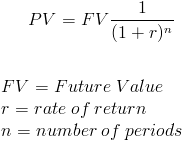Custom Search

# Present Value

PV Calculator (Click Here or Scroll Down)Present Value (PV) is a formula used in Finance that calculates the present day value of an amount that is received at a future date. The premise of the equation is that there is "time value of money".

Time value of money is the concept that receiving something today is worth more than receiving the same item at a future date. The presumption is that it is preferable to receive \$100 today than it is to receive the same amount one year from today, but what if the choice is between \$100 present day or \$106 a year from today? A formula is needed to provide a quantifiable comparison between an amount today and an amount at a future time, in terms of its present day value.

### Use of Present Value Formula

The Present Value formula has a broad range of uses and may be applied to various areas of finance including corporate finance, banking finance, and investment finance. Apart from the various areas of finance that present value analysis is used, the formula is also used as a component of other financial formulas.

#### Example of Present Value Formula

An individual wishes to determine how much money she would need to put into her money market account to have \$100 one year today if she is earning 5% interest on her account, simple interest.

The \$100 she would like one year from present day denotes the C1 portion of the formula, 5% would be r, and the number of periods would simply be 1.

Putting this into the formula, we would haveWhen we solve for PV, she would need \$95.24 today in order to reach \$100 one year from now at a rate of 5% simple interest.

##### Alternative Formula

The Present Value formula may sometimes be shown asNew to Finance?